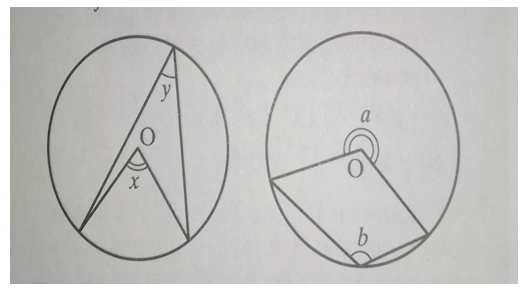### Circle Theorem

Mathematics SSS 2 Second Term

WEEK 9

Circle Theorem

Performance Objectives

Students should be able to;

1. Prove that the angle which an arc suspends at the centre is twice the angle it suspends at the circumference.
2. Solve Practical Problems on the theorem correctly.
3. Solve Problems on angles in the same segment

Circle theorems (revision)

Content

1.  The angle that an arc of a circle subtends at the centre is double that which it subtends at any point on the remaining part of the circumference.In the diagram above x = 2y

a = 2b

View More